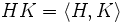# Equivalence of definitions of permuting subgroups

Suppose$G$ is a group, and$H,K$ are subgroups of$G$. Then, the following are equivalent:
1.$HK$ (the product of subgroups)is a subgroup
2.$HK = KH$
3.$HK = \langle H, K \rangle$
4.$HK \subseteq KH$
5.$KH \subseteq HK$